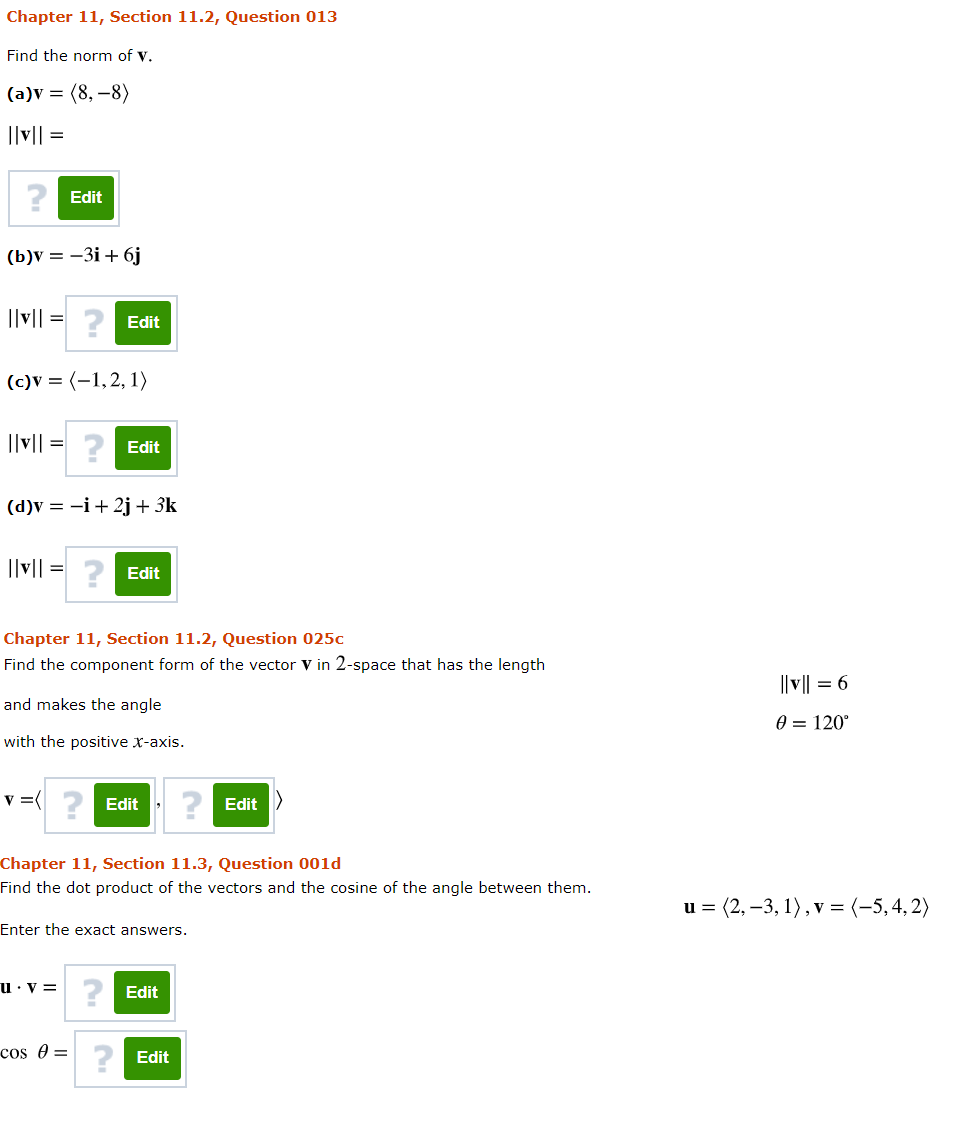# Question Chapter 11, Section 11.2, Question 013 Find the norm of v. (a)v = (8,-8) Ilv/l = ? Edit (b) = -3i+ 6j Ilvl = Edit (c)v = (-1,2,1) Ilv/l = Edit (d)v = -i +2j + 3k Ilvl = Edit Chapter 11, Section 11.2, Question 025c Find the component form of the vector V in 2-space that has the length l|v|| = 6 and makes the angle O = 120° with the positive X-axis. v = Edit Edit Chapter 11, Section 11.3, Question 0010 Find the dot product of the vectors and the cosine of the angle between them. u = (2, -3,1), v = (-5,4, 2) Enter the exact answers. u.V= Edit cos Ꮎ = ? Edit